# SPSS AMOS Moderation Analysis with Categorical Moderator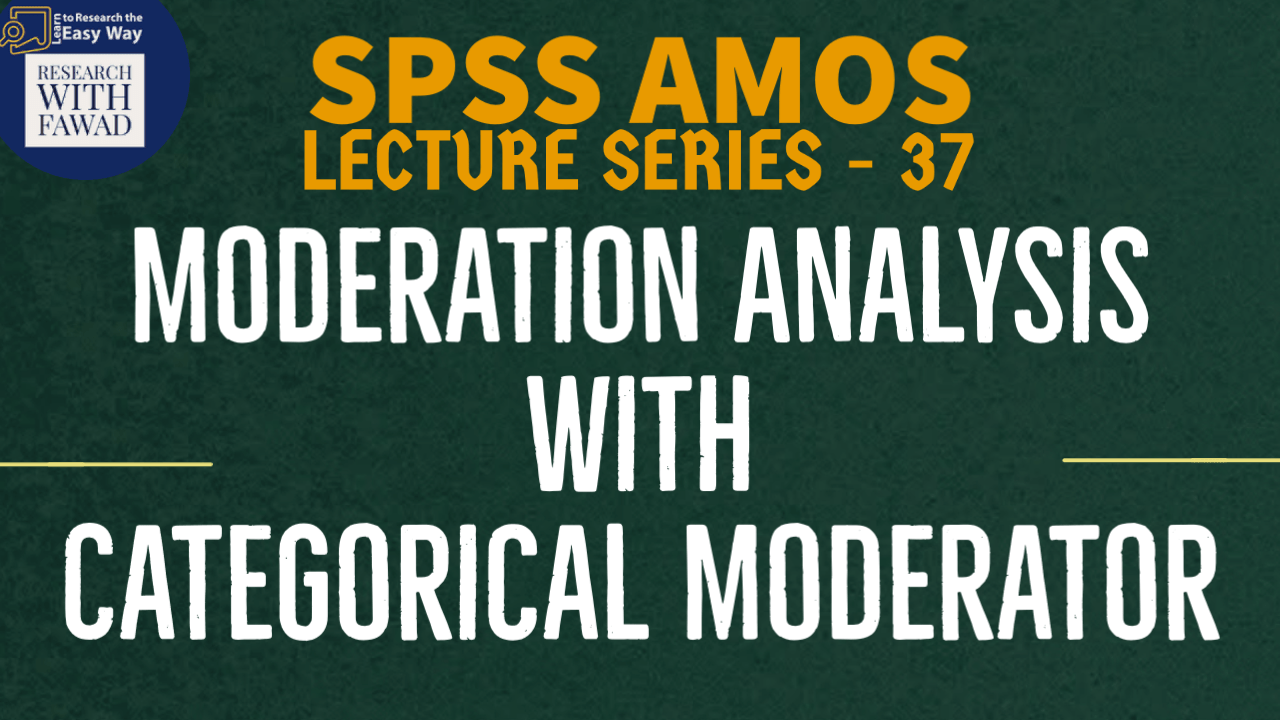### Moderation Analysis in SPSS AMOS

The Focus of the video tutorial is guiding how to perform Moderation Analysis with categorical variables in SPSS AMOS.

## Concept of Moderation

• You can use a categorical variable as a moderator in AMOS. The way to test a categorical moderator is the exact same process used to perform a two-group analysis that was discussed in earlier videos (Find below the link).
• For instance, let’s say we have a categorical moderator type of bank.
• This categorical variable is proposed to moderate the relationship from Organizational Commitment (OC) to Collaborative Culture (CC). In essence, we are hypothesizing that OC will have a stronger relationship to CC in Private Sector Banks in comparison to Public Sector Banks
• To test this moderator, we will set up a two-group analysis in AMOS where we will form two groups and then label all the parameters differently across the groups. For a detailed explanation on two group analysis, see videos shared in description.

## Test for Moderation

• Once we have labeled all the parameters for each group, we are ready to specify the relationships that need to be tested across the groups.
• AMOS will initially give you different potential models that will constrain different aspects of the model to be equal across the groups and compare this to the unconstrained model.
• We need to create a new model comparison (we can call it “Constrain 1”) because we are concerned only if the relationship from Organizational Commitment (OC) to Collaborative Culture (CC) is significantly different across the categories of the moderator.
• We are going to constrain that one path in the model and compare the results to the unconstrained model and initially see if a chi-square difference is significant across the groups.
• In the labeled model in Figure, the path we will focus in on is b3_1 (OC to CC).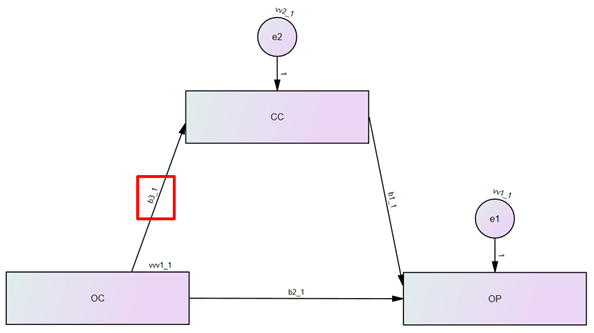• The “Constrain 1” model test is going to constrain the b3_1 (Public) relationship to the b3_2 (Private) relationship. No other model comparisons are of interest at this point.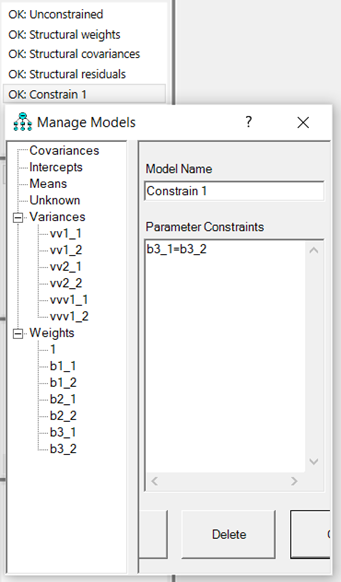• After forming the new comparison model, we are ready to run the analysis. After running the analysis, the first area in the output we want to examine is the “Model Comparison” link.
• This will give us the chi-square difference across the groups for the relationship we are testing. The results of the test show us that the “Constrain 1” model comparison had a chi-square difference of 5.421, which is significant p = .020.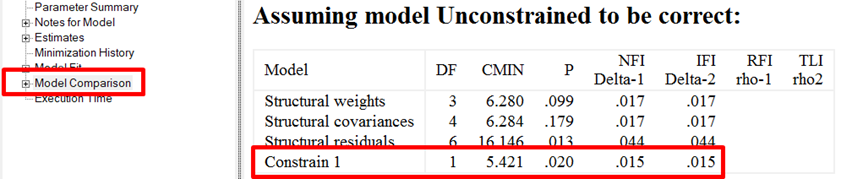• We now know the relationship is different between the groups, but I do not know if a specific category is strengthening/weakening a relationship compared to another category.
• To find out this information, we need to go to the “Estimates” link in the output and examine the regression coefficients across the groups.
• In the Estimates link, you  need to select the “Public” group and then examine the OC to CC relationship. The relationship is significant with an unstandardized regression weight of .446.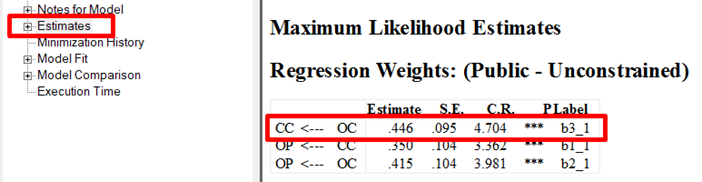• If you now select the “Private” group, you see the OC to OP relationship is significant, but the unstandardized regression weight is much higher (.695). Thus, we can conclude that Private banks have a stronger influence on the Organizational Commitment to Collaborative Culture than the Public Sector Banks.
• Our chi-square difference test shows us that the moderator is significant, and the examination of the regression coefficients lets us know how the moderator influences the specified relationship.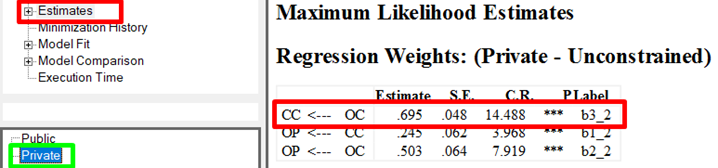•

## Moderation with Interaction

• For a continuous independent variable and a categorical moderator variable, moderation means that the slope of the relationship between the independent and dependent variable differs across the groups represented by the categorical moderator variable.

## Slop Analysis

• The results revealed a significant moderating role of Type of Bank on the relationship between OC and Collaborative Culture. The plot shows a steeper and positive gradient for private sector banks as compared to public sector banks.
• Thus, this shows that the impact of OC in fostering collaborative culture is stronger in private banks as compared to public sector banks.

## Reference

Collier, J. E. (2020). Applied structural equation modeling using AMOS: Basic to advanced techniques. Routledge.